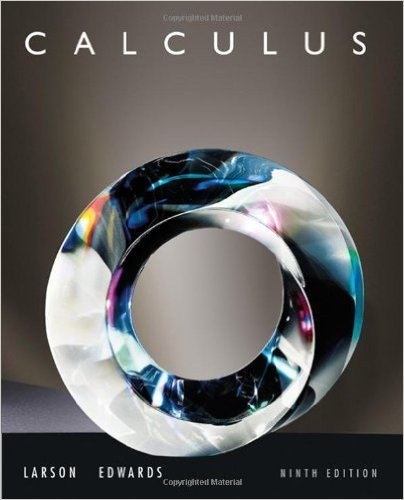×
×

# Solutions for Chapter 5: Logarithmic, Exponential, and Other Transcendental Functions## Full solutions for Calculus | 9th Edition

ISBN: 9780547167022Solutions for Chapter 5: Logarithmic, Exponential, and Other Transcendental Functions

Solutions for Chapter 5
4 5 0 269 Reviews
21
4
##### ISBN: 9780547167022

Since 97 problems in chapter 5: Logarithmic, Exponential, and Other Transcendental Functions have been answered, more than 60945 students have viewed full step-by-step solutions from this chapter. This expansive textbook survival guide covers the following chapters and their solutions. This textbook survival guide was created for the textbook: Calculus , edition: 9. Chapter 5: Logarithmic, Exponential, and Other Transcendental Functions includes 97 full step-by-step solutions. Calculus was written by and is associated to the ISBN: 9780547167022.

Key Calculus Terms and definitions covered in this textbook
• Characteristic polynomial of a square matrix A

det(xIn - A), where A is an n x n matrix

• Equation

A statement of equality between two expressions.

• Expanded form of a series

A series written explicitly as a sum of terms (not in summation notation).

• Extraneous solution

Any solution of the resulting equation that is not a solution of the original equation.

• Line of symmetry

A line over which a graph is the mirror image of itself

• Multiplication principle of probability

If A and B are independent events, then P(A and B) = P(A) # P(B). If Adepends on B, then P(A and B) = P(A|B) # P(B)

• Multiplication property of inequality

If u < v and c > 0, then uc < vc. If u < and c < 0, then uc > vc

• nth root of unity

A complex number v such that vn = 1

• Opens upward or downward

A parabola y = ax 2 + bx + c opens upward if a > 0 and opens downward if a < 0.

• Opposite

See Additive inverse of a real number and Additive inverse of a complex number.

• Outliers

Data items more than 1.5 times the IQR below the first quartile or above the third quartile.

A function that can be written in the form ƒ(x) = ax 2 + bx + c, where a, b, and c are real numbers, and a ? 0.

• Random numbers

Numbers that can be used by researchers to simulate randomness in scientific studies (they are usually obtained from lengthy tables of decimal digits that have been generated by verifiably random natural phenomena).

• Reflection through the origin

x, y and (-x,-y) are reflections of each other through the origin.

• Riemann sum

A sum where the interval is divided into n subintervals of equal length and is in the ith subinterval.

• Right-hand limit of ƒ at x a

The limit of ƒ as x approaches a from the right.

• Second

Angle measure equal to 1/60 of a minute.

• Stretch of factor c

A transformation of a graph obtained by multiplying all the x-coordinates (horizontal stretch) by the constant 1/c, or all of the y-coordinates (vertical stretch) of the points by a constant c, c, > 1.

• Symmetric property of equality

If a = b, then b = a

• x-coordinate

The directed distance from the y-axis yz-plane to a point in a plane (space), or the first number in an ordered pair (triple), pp. 12, 629.

×